### Home > PC > Chapter 7 > Lesson 7.3.2 > Problem7-109

7-109.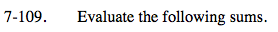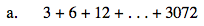Geometric Series:

$S=\frac{a(r^{n}-1)}{r-1}$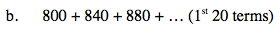Arithmetric Series:

$S=\frac{n(a_{1}+a_{n})}{2}$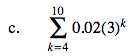Use your Graphing Calculator Sum program.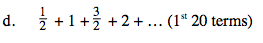Find the 20th term.
Then use the Sum formula for Arithmetic Series.

an = a1 + (n − 1)d